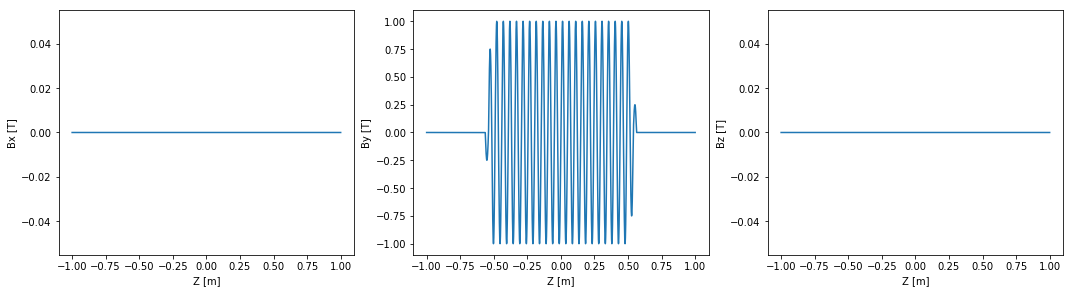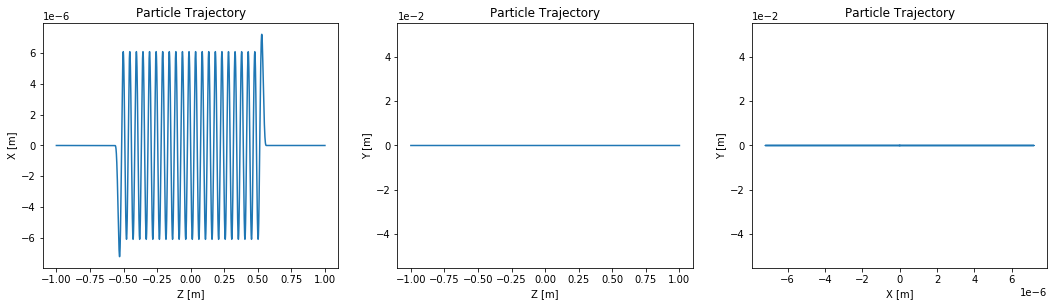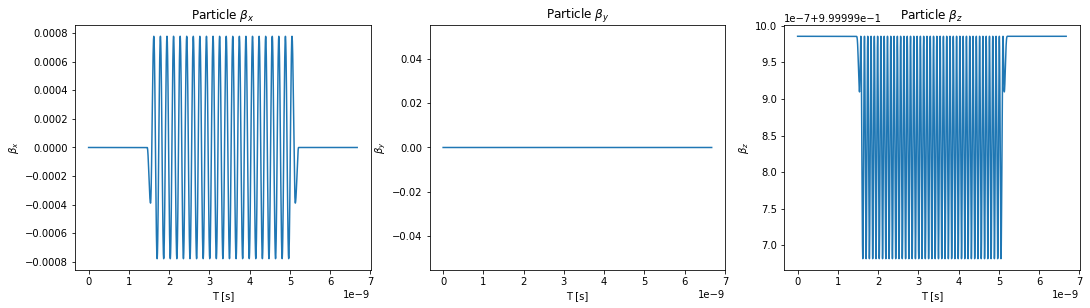# Example 002: Undulator Trajectory¶

In this example the electron trajectory in an undulator is calculated and plotted. Some attempt is made to explain each step

In :
# This has nothing to do with OSCARS, but it puts the matplotlib plots inline in the notebook
%matplotlib inline

# Import the OSCARS SR module
import oscars.sr

# Import basic plot utilities (matplotlib).  You don't need these to run OSCARS, but it's used here for basic plots
from oscars.plots_mpl import *

OSCARS v2.1.8 - Open Source Code for Advanced Radiation Simulation
Brookhaven National Laboratory, Upton NY, USA
http://oscars.bnl.gov
oscars@bnl.gov

In :
# Create a new OSCARS object.  Default to 8 threads and always use the GPU if available


## Create the undulator field¶

Here we create the undulator field. Here bfield represents maximum magnetic field [$B_x, B_y, B_z$]. The period is also in vector form which allows you to orient the axis of the undulator in any arbitrary direction. The number of periods is given by nperiods. This is the number of FULL periods. A terminating field of 1 period length is added to each side in addition to nperiods. Typically clear_magnetic_fields() is called before adding a field in notebooks only to save time when making changes and rerunning sections of the code so it is not strictly necessary.

In :
# Clear any existing fields (just good habit in notebook style) and add an undulator field
osr.clear_bfields()
osr.add_bfield_undulator(bfield=[0, 1, 0], period=[0, 0, 0.049], nperiods=21)

In :
# Just to check the field that we added seems visually correct
plot_bfield(osr)Here we add a particle beam making use of some of the defaults, namely:

* type='electron'
* t0=0
* d0=[0, 0, 1]



One must specify ctstartstop. This is the start and stop time of the calculation. In this example we will start the calculation at t=0 and go to t=2 (given in units of ct) since the beam is relativistic. In this example you can specify the start time as less than 0 which is useful if you want to propogate the particle backwars in time. This is useful for instance if you have a bending magnet before the undulator that you wish to include.

clear_particle_beams() is called, again for convenience, but it is not necessary.

In :
# Setup beam similar to NSLSII
osr.clear_particle_beams()
osr.set_particle_beam(x0=[0, 0, -1], energy_GeV=3, current=0.500)

# Set the start and stop times for the calculation
osr.set_ctstartstop(0, 2)


## Calculate Trajectory¶

Now we calculate the trajectory and plot it. It is enough to call calculate_trajectory(). If you are doing other calculations (flux, spectra, power density) it is not necesary to call this since it is called internally.

In :
# Run the particle trajectory calculation
trajectory = osr.calculate_trajectory()

# Plot the trajectory position and velocity
plot_trajectory_position(trajectory)
plot_trajectory_velocity(trajectory)